Courses

# Previous year Questions (2016-20) - Functions JEE Notes | EduRev

## JEE : Previous year Questions (2016-20) - Functions JEE Notes | EduRev

The document Previous year Questions (2016-20) - Functions JEE Notes | EduRev is a part of the JEE Course Maths 35 Years JEE Mains & Advance Past year Papers Class 12.
All you need of JEE at this link: JEE

Q.1. If g(x) = x2 + x - 1 and (gof) (x) = 4x2 - 10x + 5, then f (5/4) is equal to    (2020)
(a) 3/2
(b) - 1/2
(c) 1/2
(d) - 3/2

Ans. (b)
Solution.
We have
g(x) = x2 + x - 1 and (gof) (x) = 4x2 - 10x + 5
Now, g(f(x)) = 4x2 - 10x + 5 = 4x2 - 8x + 4 - 2x +1
⇒ g(f(x)) = (4 - 8x + 4x2) + (2 - 2x) - 1
⇒ g(f(x)) = (2-2x)2 + (2-2x) - 1 ⇒ f(x) = 2 - 2x
Hence,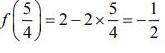Q.2. The inverse function of f(x)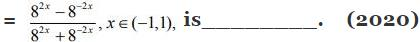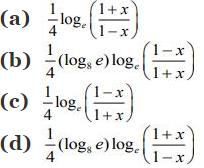Ans. (d)
Solution.
Let,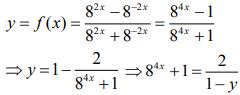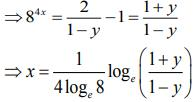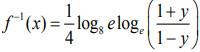Q.3. Let f: (1, 3) → R be a function defined by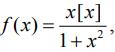where [x] denotes the greatest integer ≤ x . Then, the range of f is    (2020)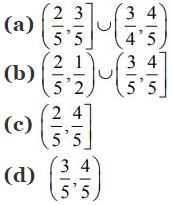Ans. (b)
Solution. We have,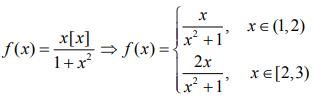Since f(x) is a decreasing function, then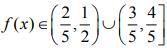Q.4. Let f and g be differentiable functions on R such that fog is the identity function. If for some a, b ∈ R g'(a) = 5 and g(a) = b then f'(b) is equal to    (2020)
(a) 1/5
(b) 1
(c) 5
(d) 2/5
Ans.
(a)
Solution.
f and g are differentiable functions on R and fog is the identity function. So,
f(g(x)) = x
⇒ f'(g(x)) . g'(x) = 1     (1)
Substituting x = a in Eq. (1), we get
f'(g(a)). g'(a) = 1
⇒ f'(b) × 5 = 1 ⇒ f'(b) = 1/5

Q.5. For x ∈ R - {0, 1}, let f1(x) = 1/x, f2 (x) = 1 - x and f3(x) = 1/1-x be three given functions. If a function, J(x) satisfies (f2oJof1) (x) = f3(x) then J(x) is equal to:    (2019)
(a) f3(x)
(b) 1/x f3(x)
(c) f2(x)
(d) f1(x)
Ans.
(a)
Solution.
The given relation is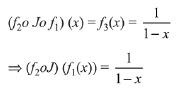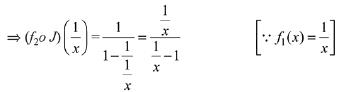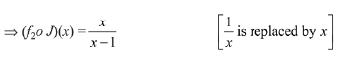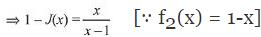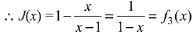Q.6. If the fractional part of the number 2403/15 is k/15, then k is equal to:    (2019)
(a) 6
(b) 8
(c) 4
(d) 14
Ans.
(b)
Solution.

2403 = 2400 · 23
= 24 × 100 · 23
= (24)100· 8
= 8(24)100 = 8(16)100
= 8(1 + 15)100
= 8 + 15μ
When 2403 is divided by 15, then remainder is 8.
Hence, fractional part of the number is 8/15
Therefore, value of k is 8

Q.7. Let A = {x ∈ R: x is not a positive integer}. Define a function f: A → R as f(x) = 2x/x - 1, then f is:    (2019)
(a) Not injective
(b) Neither injective nor surjective
(c) Surjective but not injective
(d) Injective but not surjective

Ans. (d)
Solution.
As A = {x ∈ R: x is not a positive integer}
A function f: A → R given by f(x) = 2x/x-1
f(x1) = f(x2) ⇔ x1 = x2
So, f is one-one.
As f(x) ≠ 2 for any x ∈ A ⇒ f is not onto.
Hence f is injective but not subjective.

Q.8. Let N be the set of natural numbers and two functions f and g be defined as f, g : N → N such that    (2019)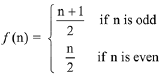and g(n) = n - (- 1)n. Then fog is:
(a) Onto but not one-one.
(b) One-one but not onto.
(c) Both one-one and onto.
(d) Neither one-one nor onto.

Ans. (a)
Solution.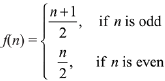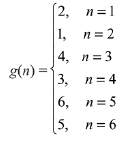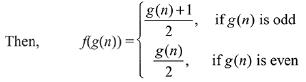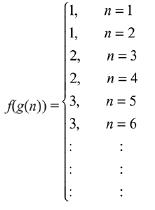⇒ fog is onto but not one - one

Q.9. Let f: R → R be defined by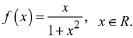Then the range of f is:    (2019)
(a) [- 1/2, 1/2]
(b) R - [-1,1]
(c) R - [- 1/2, 1/2]
(d) (-1,1) - {0}

Ans. (a)
Solution.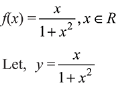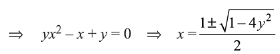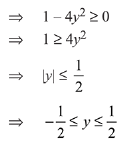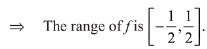Q.10. Let a function f: (0, ∞) → (0, ∞) be defined by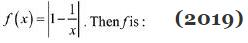(a) Not injective but it is surjective
(b) Injective only
(c) Neither injective nor surjective
(d) Both injective as well as surjective

Ans. (Bonus)
Solution.
f: (0, ∞) → (0, ∞)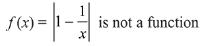∵ f(1) = 0 and 1 ∈ domain but 0 ∉ co-domain
Hence, f(x) is not a function.

Q.11. If f(x) = loge(1 - x)/(1 + x) , |x| < 1, then f(2x/1 + x2) is equal to :     (2019)
(a) 2f(x)
(b) 2f(x2)
(c) (f(x))2
(d) -2f(x)

Ans. (a)
Solution.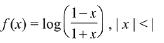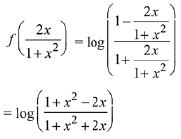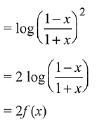Q.12. Let f(x) = ax (a > 0) be written as f(x) = f1(x) + f2(x), where f1(x) is an even function and f2(x) is an odd function. Then f1(x + y) + f1(x - y) equals:     (2019)
(a) 2 f1(x) f1(y)
(b) 2 f1(x + y) f1(x - y)
(c) 2 f1(x) f2(y)
(d) 2 f1(x + y) f2(x - y)
Ans. (a)
Solution.
Given function can be written as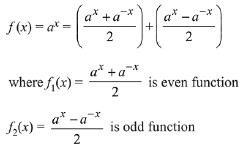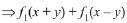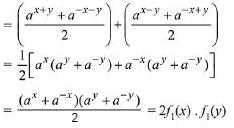Q.13. If the function f: R - {1, -1} → A defined by f(x) = x2/1 - x2, is surjective, then A is equal to:     (2019)
(a) R - {-1}
(b) [0, ∞]
(c) R- [-1, 0]
(d) R - (-1, 0]

Ans. (c)
Solution.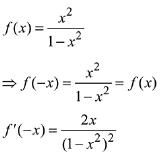∴ f(x) increases in x ∈ (0, ∞)
Also f(0) = 0 and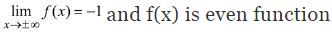∴ Set A = R - [-1, 0)
And the graph of function f(x) is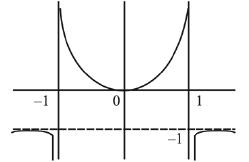Q.14.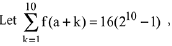where the function f satisfies f(x + y) = f(x) f(y) for all natural numbers x, y and f(1) = 2. Then the natural number 'a' is:     (2019)
(a) 2
(b) 16
(c) 4
(d) 3

Ans. (d)
Solution.
∵ f(x + y) = f(x) x f(y)
⇒ Let f(x) = tx
∵ f(1) = 2
∵ t = 2
⇒ f(x) = 2x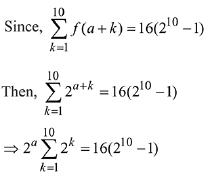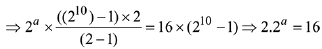⇒ a = 3

Q.15. The domain of the definition of the function(a) (-1, 0) ∪ (1, 2) ∪ (3, ∞)
(b) (-2, -1) ∪ (-1, 0) ∪ (2, ∞)
(c) (-1, 0) ∪ (1, 2) ∪ (2, ∞)
(d) (1, 2) ∪ (2, ∞)

Ans. (c)
Solution.
To determine domain, denominator ≠ 0 and x3 - x > 0
i.e., 4 - x2 ≠ 0 x ≠ ±2       ...(1)
and x (x - 1) (x + 1) > 0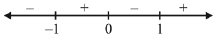x∈(-1, 0) ∪ (1, ∞)    ...(2)
Hence domain is intersection of (1) & (2).
i.e.,x ∈ (-1, 0) ∪ (1, 2) ∪ (2, ∞)

Q.16. Let f(x) = x2, x ∈ R. For any A ⊆ R, define g(A) = {x∈R: f(x) ∈ A}. If S = [0, 4], then which one of the following statements is not true?     (2019)
(a) g(f(S)) ≠ S
(b) f(g(S)) = S

(c) g(f(S)) = g(S)
(d) f (g (S)) ≠ f (S)
Ans. (c)
Solution.
f(x) = x2 ; x ∈ R
g(A) = {x ∈ R: f(x) ∈ A} S = [ 0, 4]
g(S) = {x ∈ R: f(x) ∈ S}
= {x ∈ R: 0 ≤ x2 ≤ 4} = { x ∈ R : -2 ≤ x ≤ 2}
∴ g(S) ≠ S
∴ f(g (S)) ≠ f(S)
g(f(S)) = {x ∈ R: f(x) ∈ f(S)}
= { x ∈ R : x2 ∈ S2} = { x ∈ R : 0 ≤ x2 ≤ 16}
= {x ∈ R : -4 ≤ x ≤ 4}
∴ g(f(S)) ≠ g(S)
∴ g(f(S)) = g (S) is incorrect.

Q.17. Let f(x) = loge (sinx), (0 < x < π) and g(x) = sin-1 (e-x), (x > 0). If α is a positive real number such that a = (fog)' (α) and b = (fog) (α), then:     (2019)
(a) aα2 + bα + a = 0
(b) aα2 - bα - a = 1
(c) aα2 - bα - a = 0
(d) aα2 + bα - a = - 2a
2
Ans. (b)
Solution.
f(x) = ln (sin x), g (x) = sin-1 (e-x)
⇒ f(g(x)) = ln (sin (sin-1 e-x)) = - x
⇒ f(g(α)) = - α
But given that (fog) (α) = b
- α = b and f' (g(α)) = a, i.e., a = - 1
∴ aα2 - bα - a = - α2 + a2 - (- 1)
⇒ aα2 - bα - a = 1.

Q.18. For x ∈ (0, 3/2), let f(x) = √x, g(x) = tan x and h(x) = (1-x2)/1+x2). If φ(x) = ((hof)og), (x), then φ (π/3) is equal to:     (2019)
(a) tan π/12
(b) tan 11π/12
(c) tan 7π/12
(d) tan 5π/12
Ans. (b)
Solution.
∵ φ(x) = ((hof)og)(x)
∵ φ(π/3) = h(f(g(π/3))) = h(f(√3)) = h(31/4)
= (1 - √3)/(1+√3) = - 1/2 (1 + 3 - 2√3) = √3 - 2 = -(-√3 + 2)
= -tan 15º = tan(180º - 15º) = tan(π - π/12) = tan 11π/12

Q.19. Let f(x) = 210dx + 1 and g(x) = 310x - 1. If (fog)(x) = x, then x is equal to:    (2017)
(a) (210 - 1)/(210 - 3-10)
(b) (1 - 2-10)/(310 - 2-10)
(c) (310 - 1)/(310 - 2-10)
(d) (1 - 3-10)/(210 - 3-10)

Ans. (b)
Solution.
f(g(x)) = x
f(310x - 1) = 210(310.x - 1) = x
= 1/(310 - 2-110)
210(310x - 1) + 1 = x
x(610 - 1) = 210 - 1
x = (210 - 1)/(610 - 1)    =  (1 - 2-10)/(310 - 2-10)

Q.20. The function f: N → N defined by f(x) = x - 5[x/5], where N is the set of natural numbers and [x] denotes the greatest integer less than or equal to x, is:    (2017)
(a) One-one but not onto
(b) One-one and onto
(c) Neither one-one nor onto
(d) Onto but not one-one

Ans. (c)
Solution.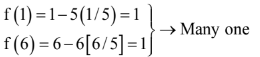f(10) = 10 – 5(2) = 0 which is not in codomain
So, the function is many one + into

Q.21. If f(x) + 2f(1/x) = 3x, x ≠ 0, and S = {x ∈ R: f(x) = f(-x)}; then S:    (2016)
(a) Is an empty set
(b) Contains exactly one element
(c) Contains exactly two elements.
(d) Contains more than two elements.
Ans.
(c)
Solution.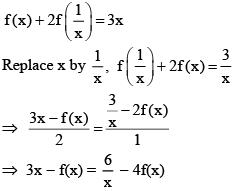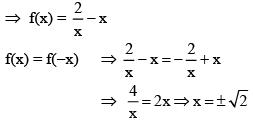Offer running on EduRev: Apply code STAYHOME200 to get INR 200 off on our premium plan EduRev Infinity!

63 docs|43 tests

,

,

,

,

,

,

,

,

,

,

,

,

,

,

,

,

,

,

,

,

,

;